Comparison of Simulation Techniques using Particle Systems

Introduction

For my final research project for Advanced Computer Graphics, I have attempted to implement a particle system that is useful for Simulations of Cloth and Rigid Body Dynamics. The implementation starts by taking an existing particle system and adding a method of integration called Verlet integration. It then extends to implement a system of springs to represent skeletons and matrices of connected particles. The system is complete when we use the simulation to compare implementations of cloth simulations.

The main source for this paper was written by Jakobsen . Jakobsen wrote a paper based on his experiences developing a physics engine and presented it to the Game Developers Conference in 2001. In the paper, Jakobsen talks about the integration technique and how to use this system to implement a believable physical reality for a video game environment.

Particle Integration

Jakobsen starts his paper by describing the integration system he uses to move the particles. Before we go into Verlet integration, let's first review Euler integration for comparison. Please note the following formulas are taken from Jakobsen's paper .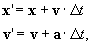The important thing to note here is that Euler depends upon both the current location of the particle and its current acceleration and velocity. In each time step, the particle's location and velocity is updated.

On the other hand, Verlet integration ignores velocity and instead rearranges Euler to use the previous and current location to move to the next location. You can see the equation here:So what are the benefits and downsides of using Verlet over Euler? First, you have one less multiplication step each timestep. In fact, the timestep squared could potentially be cached and used over and over. Also, Verlet doesn't depend upon a velocity vector so it's possible to move the particle to satisfy some constraints and not have to worry about affecting future iterations as much. On the other hand, Euler may provide a better approximation since energy could potentially leave the system of Verlet. However, Jakobsen says that a small amount of drag can be added to the system by moving to a formula of x' = 1.99x - 0.99x* + a* x Dt2.

We'll conclude this section with a diagram of our results. It can be seen in this simulation that the white particles are a result of Verlet integration whereast the magenta particles are using Euler. As you can see, the Verlet particles do not fall as far as Euler does. At this time I'm not sure exactly why this occurs, but it could be an implementation specific issue. It could also be related to the fact that the integration is losing energy. It can also be seen that the Verlet particles do not reach the bottom of the screen as fast as the Euler particles do. The diagram shows particles being generated in the upper left of the window and being shot out forward and then gravity will take over.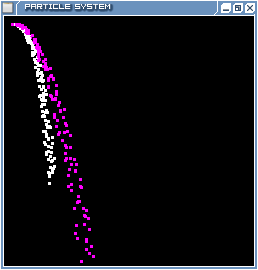Euler and verlet integration

Spring implementation

When researching this subject in relationship to Rigid body dynamics, I also was pointed towards a paper by Provot  that discusses cloth dynamics in a mass-spring system. Jakobsen himself mentions that cloth systems use springs. As a result, the natural thing to do next would be to implement a system that supports springs. Thus, we define a brief file format that allows for the pregeneration of particles (unlike the figure above) and the definitions of springs with a given rest length and stiffness constant. This stiffness determines how willing the spring is to stretch. If the stiffness is high, the spring will not want to be too far from its rest length. As the stiffness decreases, the spring will be more tolerant to deviations from the spring length.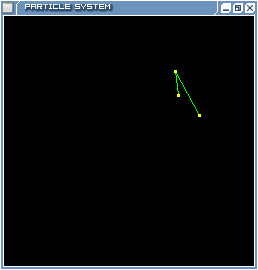Verlet integration using Jakobsen's method

When creating the springs, we must consider what kind of a system we are trying to replicate. For a typical spring system, stiffness may not be important and may vary widely among the springs. However, for rigid bodies and for cloth, we want the stiffness to be a bit high in order for the material to maintain its shape and yet still be tolerant of stretching that may occur in animation. Provot discusses an interesting fact that he found in his research. As the stiffness of the spring system is increases, the timestep interval must decrease. That is, in order to keep the system accurate, we need to ensure that we lower the timestep in order to keep the system stable. Otherwise great error may enter the system.

Constraint Satisfaction

A more important fact that we must talk about is the actual implementation of the springs themselves. How do we ensure in each timestep that the springs are correctly simulating the cloth?

Provot decides to implement a correction function that is run alongside the actual particle integration. It should be noted that he uses the Euler method for his integration. Provot runs an integration step followed by several iterations of corrections to ensure that his system is happy. While he is vague as to a specific number of timesteps (saying that it needs to be mathematically proven later), he cites the typical time to converge as 5 iterations of correction. The actual correction is quite simple. For each spring in the system, he asks them if they are happy with their current length. If the length is 10% greater than the rest length, the spring is unhappy and must be corrected to the actual rest length. Otherwise, the spring is skipped. Convergence occurs when the springs are all happy. In our implementation, we set a high limit of 10 correction iterations, however on average it could take between 3 and 7 iterations to be satisfied. This is mostly likely because there are only a few particles in our system whereas Provot used many particles in his research.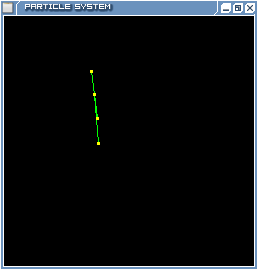Euler integration with the Provot method

Jakobsen, on the other hand, takes the system he has developed and says that we basically want springs that have infinite stiffness but are still implemented as springs so that they can be a little flexible. He terms these springs sticks because they basically have a desired length and no stiffness. Where Provot calculates the spring forces as part of the acceleration of a particle, Jakobsen treats the spring rest lengths as constraints in a very large linear program that is trying to minimize error in as fast time as possible. So, Jakobsen first iterates over all particles once to move them according to the Verlet integration and adds forces such as gravity at that step, and then moves to his constraint satisfaction step where he iterates over all springs and satisifies their constraints whether they are at the rest length or not. That is, where Provost asks the spring whether it wants to be moved, Jakobsen moves the spring without asking. An interesting point he makes is that the springs do not necessarily have to be correct and at their perfect rest length because Verlet will take care of them within a few time steps. As a result, he only iterates over the springs once versus Provot's 5 times. It could be said that Jakobsen's algorithm is bound at O(n + m) where n is the number of vertices and m is the number of springs.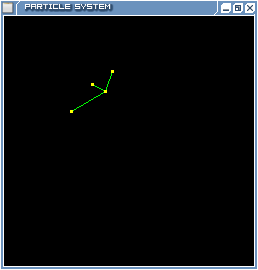Verlet integration with the Provot method

Issues

The final system works fairly well, however the actual implementation creates a few problems. In order to acurrately model the results of an animated system in real time, we decided to allow one point to oscillate and the rest would be attached from there. One problem with the current implementation is that for the Verlet implementation of the Jakobsen model, the particle attached to the fixed point tends to move directly to the fixed particle that is moving. Also, Euler integration doesn't work with the Jakobsen model.

Sources

1. Jakobsen, Thomas. "Advanced Character Physics." http://teknikus.dk/tj/gdc2001.htm.
2. Provot, Xavier. "Deformation Constraints in a Mass-Spring Model to Describe Rigid Cloth Behavior." In Proc. Graphics Interface '95, pp 147-154. 1995.